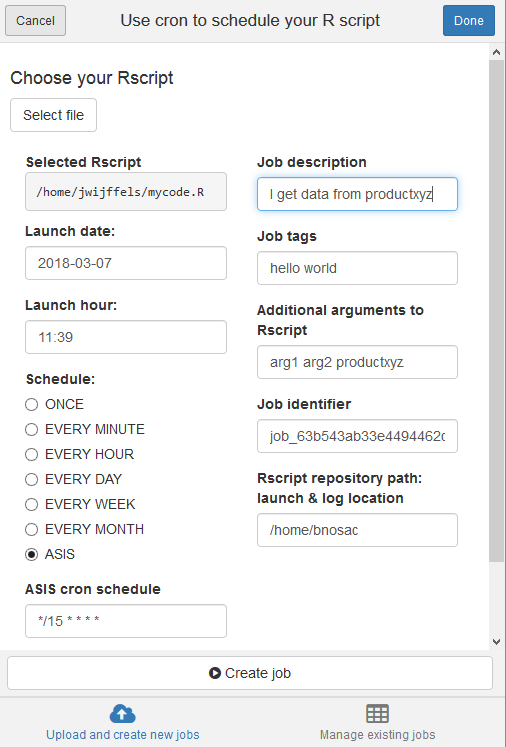Schedule R scripts/processes with the cron scheduler. This allows R users working on Unix/Linux to automate R processes on specific timepoints from R itself. Mark that if you are looking for a Windows scheduler, you might be interested in the R package taskscheduleR available at https://github.com/bnosac/taskscheduleR

## Basic usage

This R package allows to

• Get the list of scheduled jobs
• Remove scheduled jobs

• A job is basically a script with R code which is run through Rscript
• You can schedule tasks 'ONCE', 'EVERY MINUTE', 'EVERY HOUR', 'EVERY DAY', 'EVERY WEEK', 'EVERY MONTH' or any complex schedule
• The task log contains the stdout & stderr of the Rscript which was run on that timepoint. This log can be found at the same folder as the R script

When the task has run which is scheduled with the RStudio addin, you can look at the log which contains everything from stdout and stderr. The log file is located at the directory where the R script is located.

## Get started

First make sure cron is installed and the cron daemon is running. On Debian this is done as follows.

``````sudo apt-get update
sudo apt-get install -y cron
sudo cron start
``````

Next, we can start on scheduling jobs

``````library(cronR)
f <- system.file(package = "cronR", "extdata", "helloworld.R")
cmd <- cron_rscript(f)
cmd

cron_add(command = cmd, frequency = 'minutely', id = 'test1', description = 'My process 1', tags = c('lab', 'xyz'))
cron_add(command = cmd, frequency = 'daily', at='7AM', id = 'test2')
cron_njobs()

cron_ls()
cron_ls()

cmd <- cron_rscript(f, rscript_args = c("productx", "arg2", "123"))
cmd
cron_add(cmd, frequency = 'minutely', id = 'job1', description = 'Customers')
cron_add(cmd, frequency = 'hourly', id = 'job2', description = 'Weather')
cron_add(cmd, frequency = 'hourly', id = 'job3', days_of_week = c(1, 2))
cron_add(cmd, frequency = 'hourly', id = 'job4', at = '00:20', days_of_week = c(1, 2))
cron_add(cmd, frequency = 'daily', id = 'job5', at = '14:20')
cron_add(cmd, frequency = 'daily', id = 'job6', at = '14:20', days_of_week = c(0, 3, 5))
cron_add(cmd, frequency = 'daily', id = 'job7', at = '23:59', days_of_month = c(1, 30))
cron_add(cmd, frequency = 'monthly', id = 'job8', at = '10:30', days_of_month = 'first', days_of_week = '*')
cron_add(cmd, frequency = '@reboot', id = 'job9', description = 'Good morning')
cron_add(cmd, frequency = '*/15 * * * *', id = 'job10', description = 'Every 15 min')
cron_ls()
``````

When the task has run, you can look at the log which contains everything from stdout and stderr. The log file is located at the directory where the R script is located.

``````## log file is at the place where the helloworld.R script was located
system.file("extdata", "helloworld.log", package = "cronR")
````````````install.packages('miniUI')